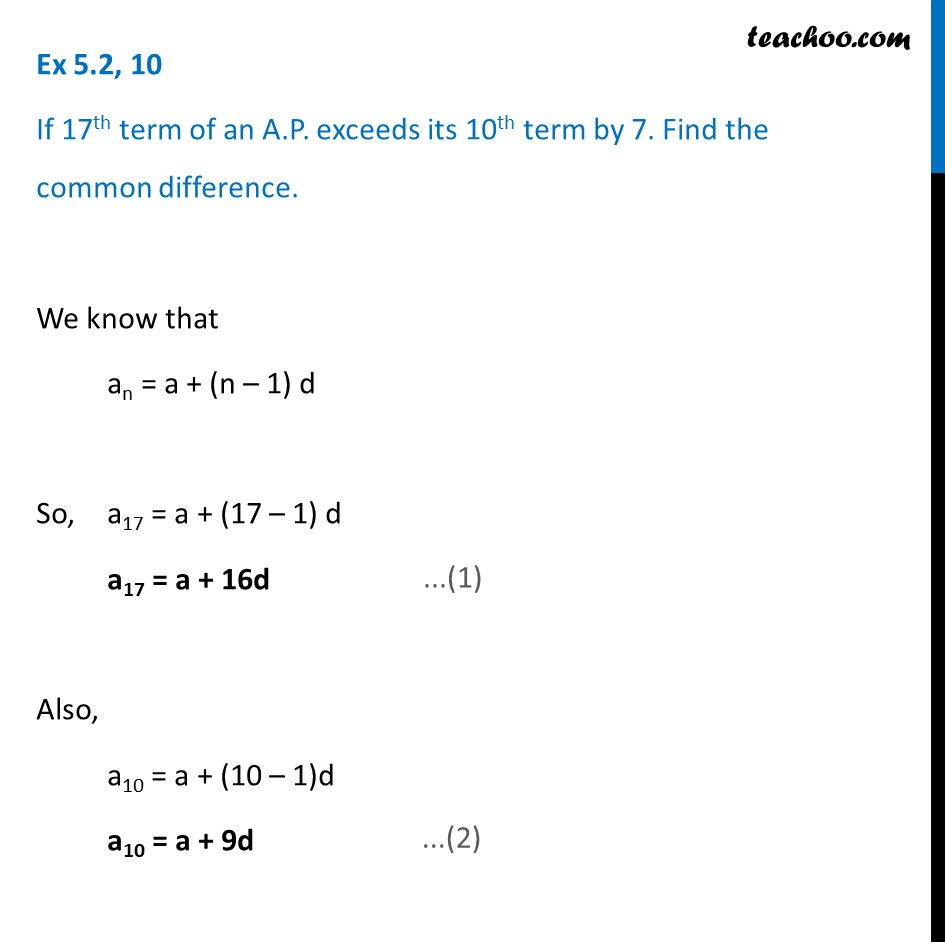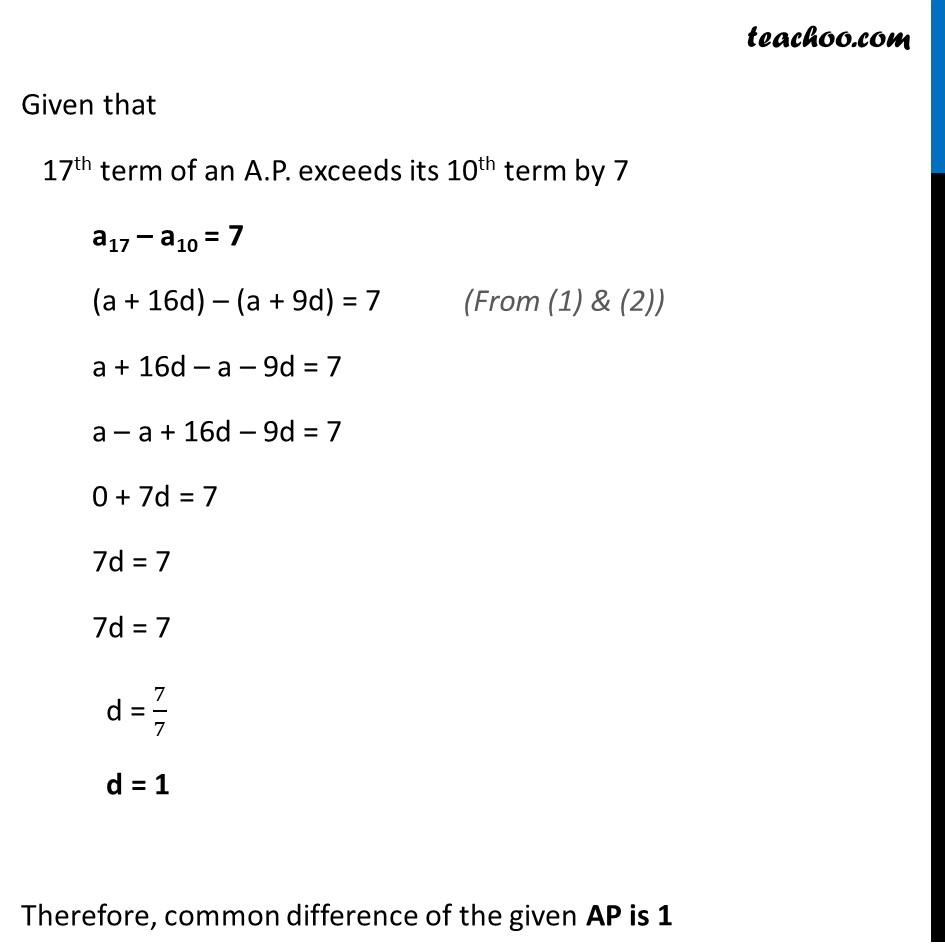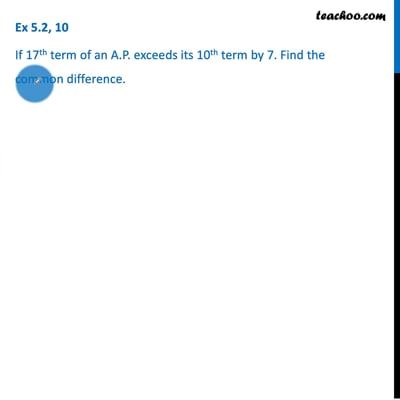Ex 5.2

Chapter 5 Class 10 Arithmetic Progressions (Term 2)
Serial order wiseThis video is only available for Teachoo black users

### Transcript

Ex 5.2, 10 If 17th term of an A.P. exceeds its 10th term by 7. Find the common difference. We know that an = a + (n – 1) d So, a17 = a + (17 – 1) d a17 = a + 16d Also, a10 = a + (10 – 1)d a10 = a + 9d Given that 17th term of an A.P. exceeds its 10th term by 7 a17 – a10 = 7 (a + 16d) – (a + 9d) = 7 a + 16d – a – 9d = 7 a – a + 16d – 9d = 7 0 + 7d = 7 7d = 7 7d = 7 d = 7/7 d = 1 Therefore, common difference of the given AP is 1 (From (1) & (2))